Homework Help Question & Answers

# Find w=a +bi = VE , where a and b are real numbers. 7601 - 11...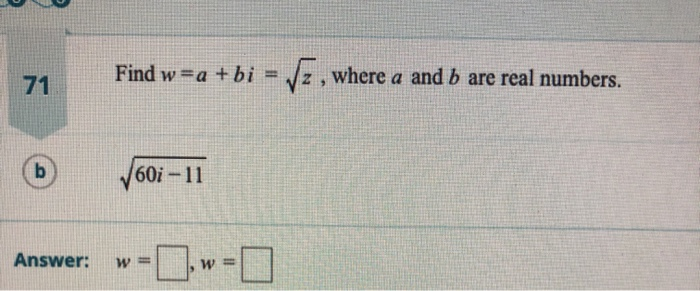Find w=a +bi = VE , where a and b are real numbers. 7601 - 11 Answer: w= w=

#### Homework Answers

Answer #1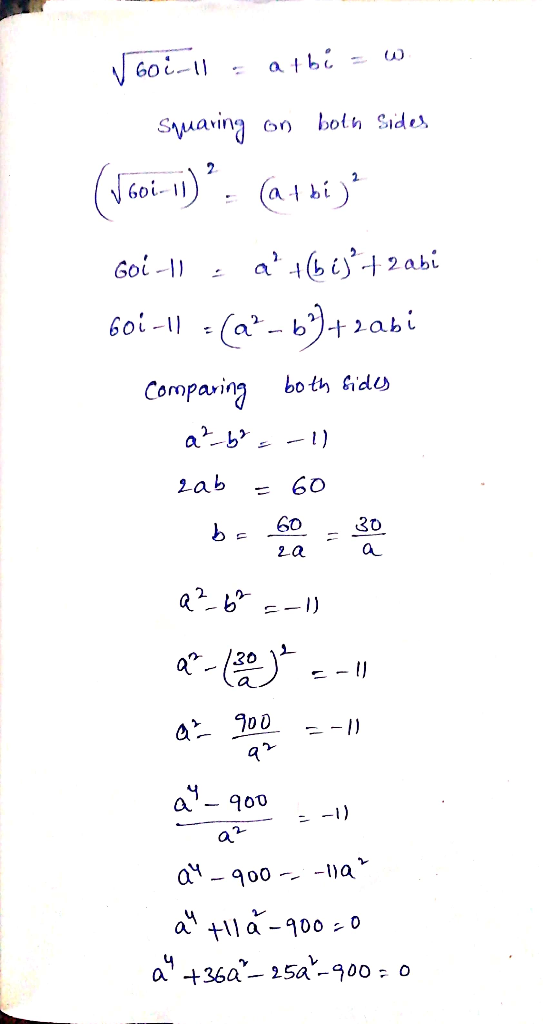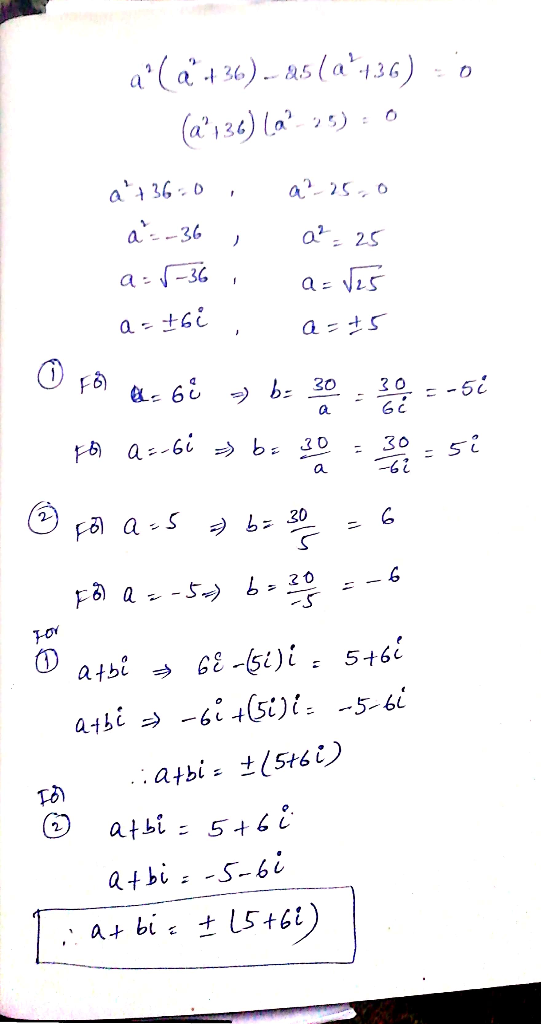Know the answer?
Your Answer:

#### Post as a guest

Your Name:

What's your source?

#### Earn Coin

Coins can be redeemed for fabulous gifts.

Not the answer you're looking for? Ask your own homework help question. Our experts will answer your question WITHIN MINUTES for Free.
Similar Homework Help Questions
• ### 1. (2 points)Let A = [~=? bi z] where a, b, and c unknown real numbers....1. (2 points)Let A = [~=? bi z] where a, b, and c unknown real numbers. Find all values a, b,and c so that A is symmetric and det(A) = 0.

• ### Perform the indicated operations and write the answer in the form a + bi, where a and b are real numbers

Perform the indicated operations and write the answer in the form a + bi, where a and b are real numbers. Problem #1 (3+2i)^2Problem #2 i^3 - i^2

• ###  (a) Find real numbers a and b such that a + bi = p.v.(-87]1/3. (b)...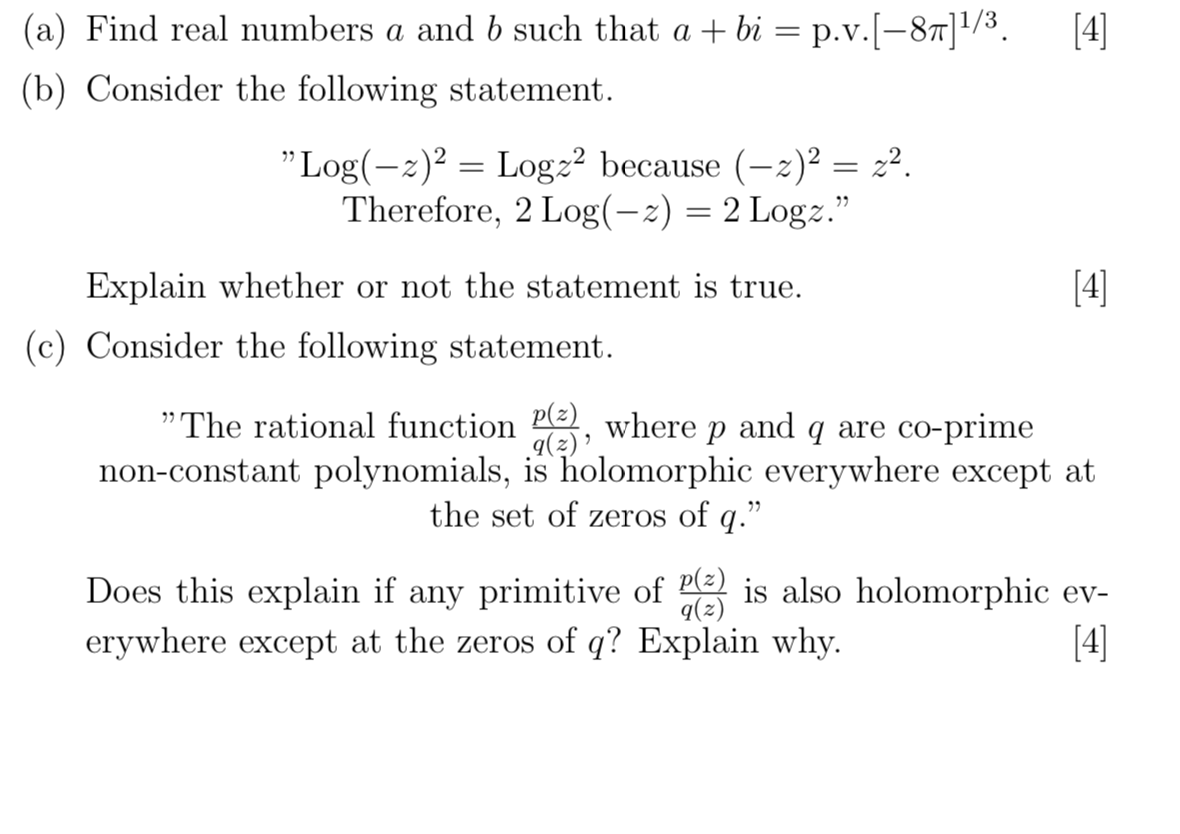(a) Find real numbers a and b such that a + bi = p.v.(-87]1/3. (b) Consider the following statement. ”Log(–z)2 = Logz2 because (-2)2 = 22. Therefore, 2 Log(-x) = 2 Logz.” Explain whether or not the statement is true.  (c) Consider the following statement. "The rational function P(3), where p and q are co-prime 9(2) non-constant polynomials, is holomorphic everywhere except at the set of zeros of q.” Does this explain if any primitive of P(z) is...

• ###  (a) Find real numbers a and b such that a + bi = p.v.(-87]1/3. (b)...(a) Find real numbers a and b such that a + bi = p.v.(-87]1/3. (b) Consider the following statement. ”Log(–z)2 = Logz2 because (-2)2 = 22. Therefore, 2 Log(-x) = 2 Logz.” Explain whether or not the statement is true.  (c) Consider the following statement. "The rational function P(3), where p and q are co-prime 9(2) non-constant polynomials, is holomorphic everywhere except at the set of zeros of q.” Does this explain if any primitive of P(z) is...

• ### 1. (a) Find real numbers a and b such that a + bi = p.v.(-87]1/3. ...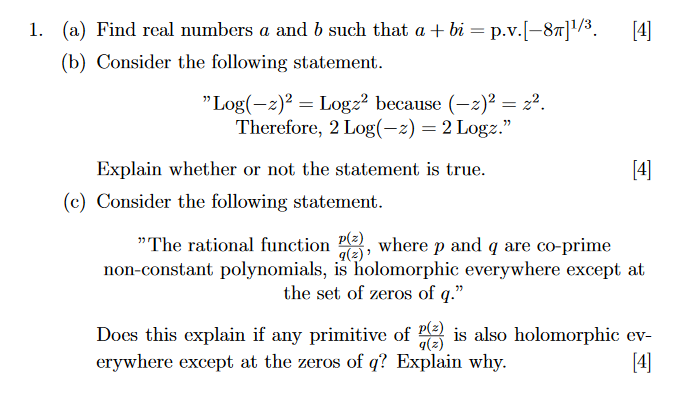1. (a) Find real numbers a and b such that a + bi = p.v.(-87]1/3.  (b) Consider the following statement. "Log(-2)2 = Logza because (-2)2 = 22. Therefore, 2 Log(-2) = 2 Logz." Explain whether or not the statement is true.  (c) Consider the following statement. ” The rational function (3), where p and q are co-prime non-constant polynomials, is holomorphic everywhere except at the set of zeros of q." Does this explain if any primitive of (...

• ###  1. (a) Find real numbers a and b such that a + bi = p.v.(-87]1/3....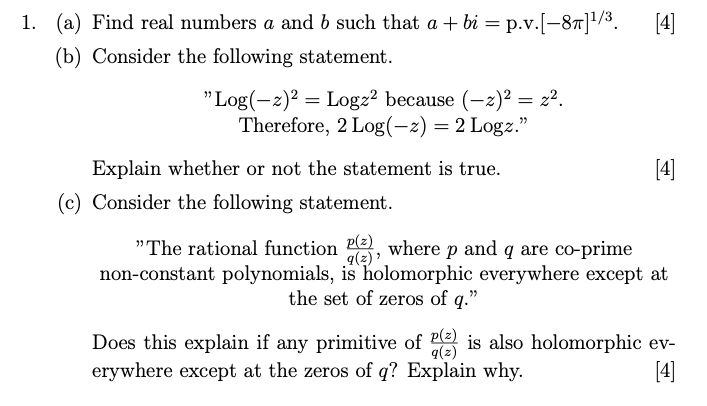1. (a) Find real numbers a and b such that a + bi = p.v.(-87]1/3. (b) Consider the following statement. "Log(-2)2 = Logz2 because (-2)2 = 22. Therefore, 2 Log(-2) = 2 Logz." Explain whether or not the statement is true.  (c) Consider the following statement. » The rational function (3), where p and q are co-prime non-constant polynomials, is holomorphic everywhere except at the set of zeros of q.” Does this explain if any primitive of (2is...

• ### Simplify and write your answer in the form of a + bi, where a and b...

Simplify and write your answer in the form of a + bi, where a and b are real numbers:   please explain.

• ### Select any imaginary number (of the form "a + bi" where a and b are non-zero real numbers), and turn it into a real number by using addition, subtraction, multiplication, division or exponentiation

Select any imaginary number (of the form "a + bi" where a and b are non-zero real numbers), and turn it into a real number by using addition, subtraction, multiplication, division or exponentiation? Did I do this correct: 2i = 2i * 2i = 2; (2i)^2 = 2i * 2i = 2 * 2 * i^2 = 4(-1) = -4

• ### 4-3i 11. (8 pts) Forz 4-3i much as possible, writin work. i and w 7- i,...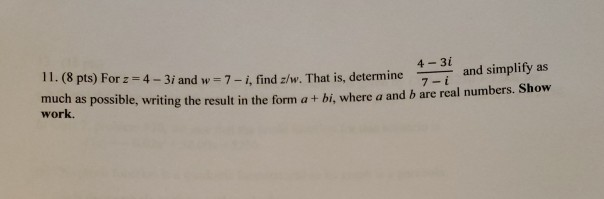4-3i 11. (8 pts) Forz 4-3i much as possible, writin work. i and w 7- i, find z/w. That is, determineand simplify as g the result in the form a + bi, where a and b are real numbers. Show

Free Homework App

Scan Your Homework
to Get Instant Free Answers
Need Online Homework Help?

Get Answers For Free
Most questions answered within 3 hours.